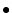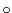## Example of Header

(1) Needed PartThe keywords for standard FITS

 ```SIMPLE = T / file does conform to FITS standard BITPIX = -32 / number of bits per data pixel NAXIS = 2 / number of data axes NAXIS1 = 512 / length of data axis 1 NAXIS2 = 512 / length of data axis 2 ```Basic information for NoRH dataInformation on observation time

 ```DATE-OBS= '1998-05-09' / TIME-OBS= '03:20:23.754' / STRT-OBS= '03:20:21.254' / END-OBS = '03:20:26.254' / JSTDATE = '1998-05-09' / JSTTIME = '12:20:23.754' / JST-STRT= '12:20:21.254' / JST-END = '12:20:26.254' / STARTFRM= 16499 / ENDFRM = 16503 / ```Information of data contents

 ```POLARIZ = 'r+l ' / ATT-10DB= '00dB ' / OBS-MODE= 'alt ' / OBS-FREQ= '17GHz ' / FRM-STAT= '1-sec obs' / DATA-TYP= 'cleaned_map' / OBJECT = 'sun ' / TELESCOP= 'radioheliograph' / ORIGIN = 'nobeyama radio obs' / ```Information of FITS header version

 ```HDRIDENT= 'HeliogFITS 2.0' / ```

(2) Ephemeris

 ```SOLR = 952.22 / optical solar radius (arcsecond) SOLP = -22.5235 / solar polar angle (degree) SOLB = -3.3307 / solar b0 (degree) DEC = 17.2807 / declination (degree) HOURA = 2270.83 / hour angle (second) AZIMUTH = 27.7610 / azimuth (degree) ALTITUDE= 70.3056 / altitude (degree) ZANGLE = 23.5280 / zenithangle (degree) PMAT1 = 0.98640 / projection matrix PMAT2 = 0.09448 / projection matrix PMAT3 = -0.04883 / projection matrix PMAT4 = 0.94981 / projection matrix ```

(3) Image coordinates

 ```CRVAL1 = 0.00 / arcsec CRVAL2 = 0.00 / arcsec CRPIX1 = 257.00 / CRPIX2 = 257.00 / CDELT1 = 4.91106 / arcsec CDELT2 = 4.91106 / arcsec CTYPE1 = 'solar-west' / CTYPE2 = 'solar-north' / ```

(4) Parameters for each synthesis programexample for Hanaoka

 ```PROGNAME= 'snap2d17 v5.1 Y. Hanaoka' / BUNIT = 'K ' / disk = 10000 K SOLR-FAC= 1.01250 / radius correction factor NFRCAL = 1 / number of calibration frames CRINPUT = -0.50 / clean criterion input MBEAMC = 'yes ' / main beam correction DISKRSTR= 'yes ' / disk restoration DDOFF1 = -12.77 / x-offset of the dirty disk DDOFF2 = 10.68 / y-offset of the dirty disk DDCORR = 0.9631 / correlation between dirty disk and model DSKBR = 37145.51 / brightness of the dirty disk CRITER = 24384.39 / clean criterion NCOMPO = 1050 / number of clean components ```example for Koshix

 ```PROGNAME= 'snap2d51_koshix_vfast' / FRAME = 16501 / ICAL = 3 / integration for calibration ITGR = 3 / integration for restoration ICLN = 3 / integration after restoration BUNIT = 'k ' / disk = 10000 K CRITER = 3000.0 / CLEAN criterion SOLR-FAC= 1.01250 / radius correction factor GAINP = 0.020 / CLEAN loop gain for estimation of sky and disk GAINE = 0.200 / CLEAN loop gain TRIM = 0.800 / CLEAN trim level NCOMPO = 66143 / number of CLEAN components DDOFF1 = 0.5 / x-offset of the dirty disk DDOFF2 = 0.9 / y-offset of the dirty disk NCLNYN = 'yes ' / negative CLEAN JCIMYN = 1 / jitter - add/sub - integration PJIMYN = 1 / projection - integration MBEAMC = 'yes ' / main beam correction ```

service@solar.nro.nao.ac.jp